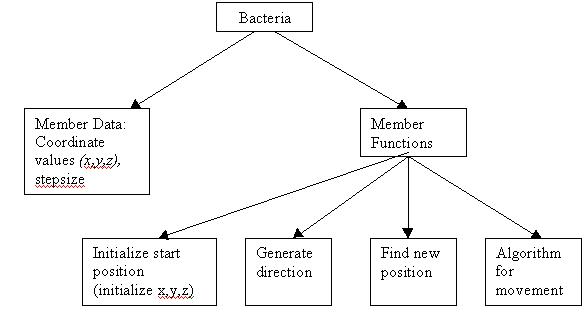Classes Explanation

A Practical use of classes:

The Problem:

Living forms (plants, animals and humans) haven’t been fully understood by man. There are many things in life that are very complex and the most complex of all is the human being. A simpler life form would be the bacteria. Nowadays, researchers are trying to mimic or imitate the behaviour of living organisms (like bacterial movement). Consider the problem of optimization. Optimization means finding the best point in a given area. How do we decide which point is best? As a simple example, consider an equation with a single variable ‘x’. Let us assume that you have to find the lowest value for that equation (the equation is also called as a function). In this case the best value means the lowest value. Let the equation be:

cost = 10*x + 100 …given that x is greater than 0 and can only take positive integer values between 1 and 100.

What value of x do you think gives the least cost?

If x=1 then cost=110; if x=2 then cost=120 and so on…

It is quite clear that x=1 will give the least cost. Hence the best point (or optimum value) is x=1.

That was very easy. The equation was very simple, involving just one variable. In real application you would have equations involving more than one variable and you will also have many conditions (the only condition we used was that x should lie between 1 and 100).

You can extend the problem to the case of two variables.

Let’s assume that we have to find the least value for a given equation that has two variables (x and y). Let the limits of x and y be between 1 to 100 (and assume that they can take only integer values). The solution space (or the area in which you have to find the best value) will be as below:It is clear from the above graph that there will be 100*100 (=10,000) possible coordinates (in other words 10,000 possible combined values for x and y). The simplest way to find the minimum value for a function involving x and y would be to substitute all the 10,000 combinations, find the cost for each combination and then choose the minimum value. This seems to be a straightforward and simple method, but you can imagine the computational time needed when the solution space increases in size. And if you include floating point values, you are going to end up with a major problem. This is why we need techniques for optimization. We need to use algorithms that can determine the best point in a given solution space as quickly as possible (i.e. the algorithm should not calculate values for each and every combination).

This is where bacterial movement comes into the picture. You might have heard of the E.Coli bacteria. This bacterium lives in the human body and has a particular method of movement. It keeps searching for areas where there is more food (or nutrition). Assume that the bacteria is initially at some place in your intestine. It will take a small step in one particular direction. If the new place has more nutrition than the previous position, then it will continue to move in the same direction. Otherwise, it will take a different direction and proceed. Suppose the new position has more nutrition, it will take another step in the same direction. Again if the next position is favourable, it will proceed in the same direction. This movement is a very simplified version of the actual movement (there are more complicated steps involved in bacterial movement but we will not deal with them here). Now you can apply the same bacterial movement algorithm to the problem of optimization. The advantage is that you won’t be computing values for each and every point in the solution space (i.e. we will program in such a way that after a particular number of iterations the program will terminate). There are many other organisms in nature which have different ways of movement and this could also be applied to the problem of optimization (in fact algorithms relating to ants, bees and snakes have been developed already).

So, where do we make use of classes and objects?

Bacteria is a general category and it forms the class. All types of bacteria will have some stepsize (stepsize refers to the length of the stride taken by the bacteria in moving once) and some particular direction as well (bacteria are in three-dimensional space and they are free to take any direction). Thus, these features are common to all bacteria. We can then model bacteria as a class. This class could contain the following functions:

• To initialize the starting position of the bacteria. Every bacterium when it is created will have a particular position in 3 dimension. This has to be specified by three variables: x,y and z.
• To generate the direction in which the bacteria should move (in mathematics this is called the unit vector).
• To find the new position after the bacteria moves.
• An algorithm to decide whether to move to the new position or not (move only if new place is better than the present one).

Every instance created from the class ‘bacteria’ is an object. Each object will have a different starting position (decided randomly) and each bacteria will move in different directions (i.e. each one will try to find the best point).

Thus, we can create 10 objects of type ‘bacteria’ and make each bacteria search the solution space. After some particular number of iterations, we can stop the bacterial movement and find out the positions of each of the objects.Continue with this explanations

or go back to Contents page.